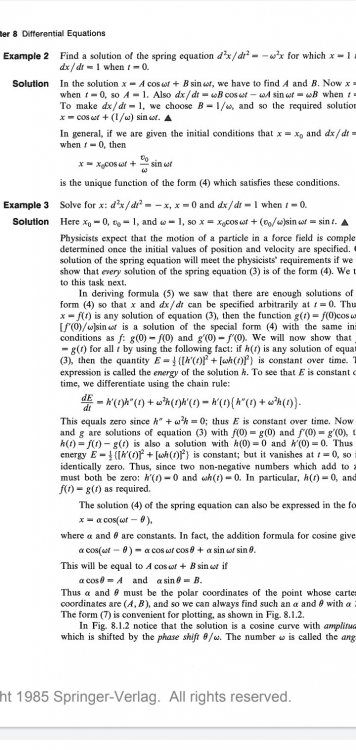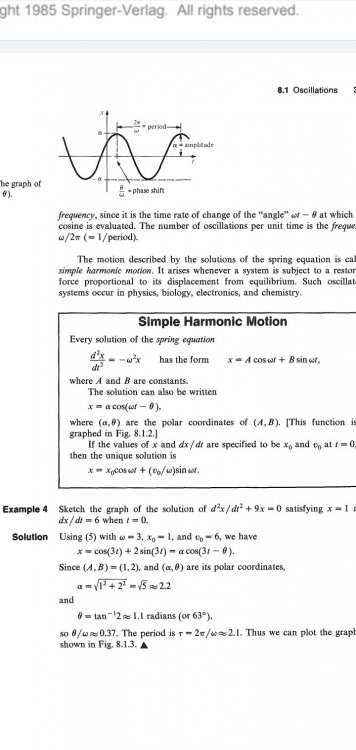Problem with differential equations

Recommended Posts

In Example 3 and Example 4 what is the meaning of omega? Why is it 1 and 3 respectively? I don't know how these results are obtained. I differentiated the expression above Example 3 and filled in with the given information in Example 3 but it didn't make anything clear.

Thanks.Share on other sites

In mathematical terms, Omega is the same to-be-determined variable as in Example 2. In terms of Physics, it is the https://en.wikipedia.org/wiki/Angular_frequency.

Example solution for Example 3:

If I take x = x0 cos (wt) + v0/w sin (wt) and plug in x(t=0) = 0, then I get the condition

x0 cos (0) + v0/w sin(0) = 0.

Hence, x0 = 0.

The first derivative of the function is dx/dt = w x0 (-sin(wt)) + v0 cos (wt).

Plugging in the constraint dx/dt (x=0) = 1 yields

w x0 (-sin(0)) + v0 cos (0) = 1.

Hence, v0 = 1.

The 2nd derivative of the function is d^2 x/dt^2 = -w^2 x0 cos(wt) - w v0 sin(wt).

That one is a bit tricky, but by comparison you will see that this is -w^2 * (original function). So this satisfies the condition d^2 x/dt^2 = -x if w^2 = 1. I don't know why w=-1 is not considered here; maybe I missed something.

Hope that helps. If so, it would be nice to hear what the actual step was that you got stuck on.

Share on other sites

52 minutes ago, timo said:

Hope that helps. If so, it would be nice to hear what the actual step was that you got stuck on.

Thanks for your help. I just wanted to stick to the book procedure but sometimes the book makes it harder than it really is, this has happened before.

Share on other sites

• 2 weeks later...

Actually, the book specifically tells you what omega is in problem 2!  There the equation is d^2x/dt^2= omega x.  In problem 3 the equation is d^2x/dt^2= -x.  Comparing that to problem 2 you should immediately see that problem 3 is the same as problem 2 with omega= 1.

Edited by HallsofIvy
Share on other sites

1 hour ago, HallsofIvy said:

Actually, the book specifically tells you what omega is in problem 2!  There the equation is d^2x/dt^2= omega x.  In problem 3 the equation is d^2x/dt^2= -x.  Comparing that to problem 2 you should immediately see that problem 3 is the same as problem 2 with omega= 1.

" the book specifically tells you what omega is in problem 2!  There the equation is d^2x/dt^2= omega x."

Seems to me that example 2 starts with

$\frac{{{d^2}x}}{{d{t^2}}} = - {\omega ^2}x$

Did you know that you can expand the attachment so that you don't need a magnifying glass to read it.Edited by studiot
Share on other sites

With my eyes, that probably wouldn't help!  But thanks for the correction.

Create an account

Register a new account